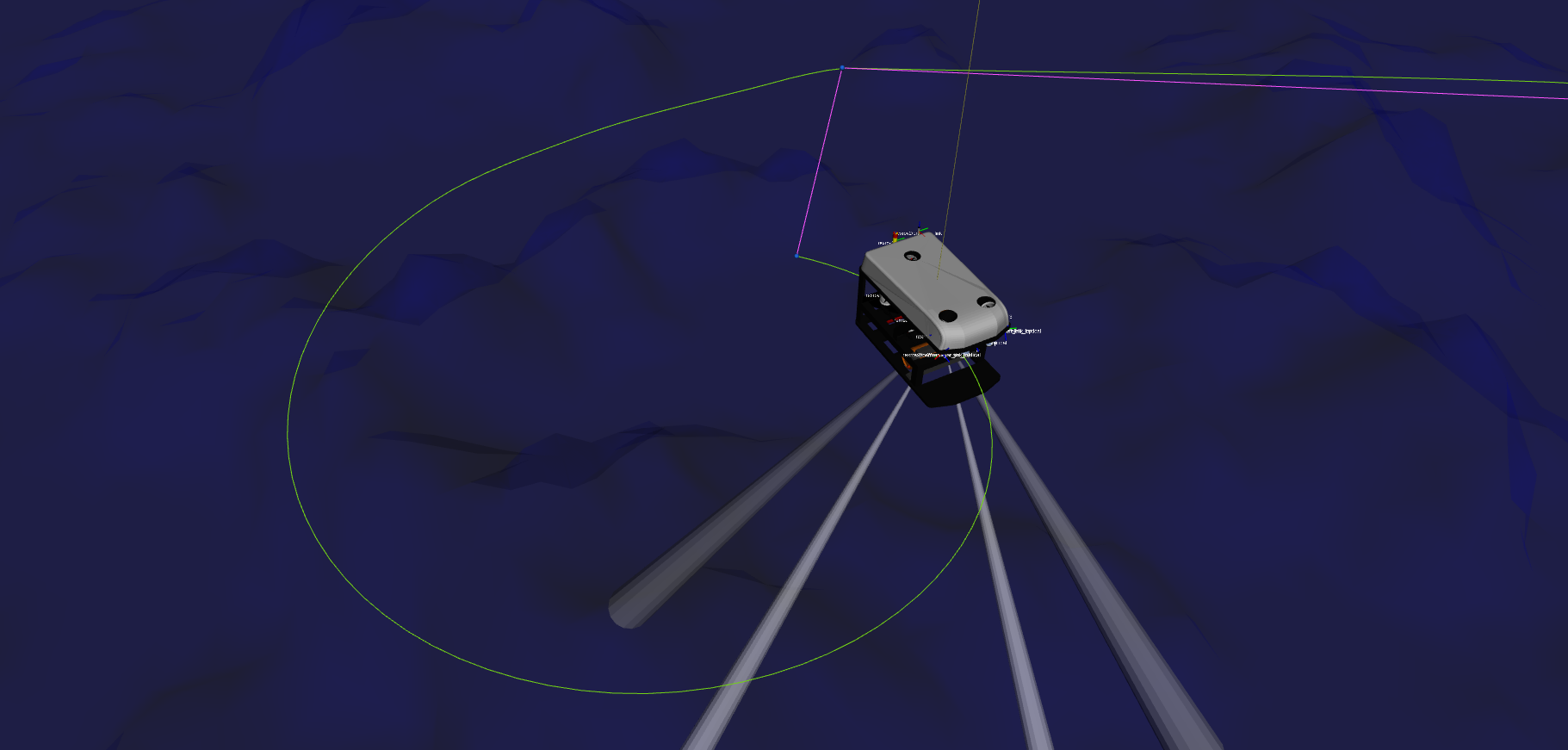# Path and trajectory generators

The generation of paths and trajectories in this package are mostly waypoint-based. The reference to the controllers are computed by using path interpolators and then finite differentiation for velocity and acceleration set-points, in case they are desired.

For the path interpolation to be possible, two Python modules are included in the uuv_trajectory_control package: uuv_waypoints and uuv_trajectory_generator.

#### Path generation¶

##### Path segments¶

The interpolators shown in the next section make use of line or curve segments that are generated in a way to have a final smooth path (with exception of the linear interpolator).

All segments are represented by parametric equations in order to allow easy computation of paths and trajectories for any range of time.

###### Line segment¶

The line segment simply connects two points in space and allows computation of any points belonging to the line.

###### Helical segment¶

The helical curve segment can be parametrized to generate waypoints that when interpolated form a helical path.

Note

An example of the use of the HelicalSegment class from uuv_trajectory_generator Python module can be seen in this Jupyter notebook

Example

To see a sample of a helical path being followed by a vehicle, you can start an ROV model with a trajectory tracking controller, such as the following from the rexrov2 package.

roslaunch rexrov2_gazebo start_demo_pid_controller.launch


To generate a helical path with two turns, run the following

roslaunch uuv_control_utils start_helical_trajectory.launch uuv_name:=rexrov2 n_turns:=2


This example uses the cubic interpolator on the generated waypoints. The resulting path can be seen as the green line in the image below.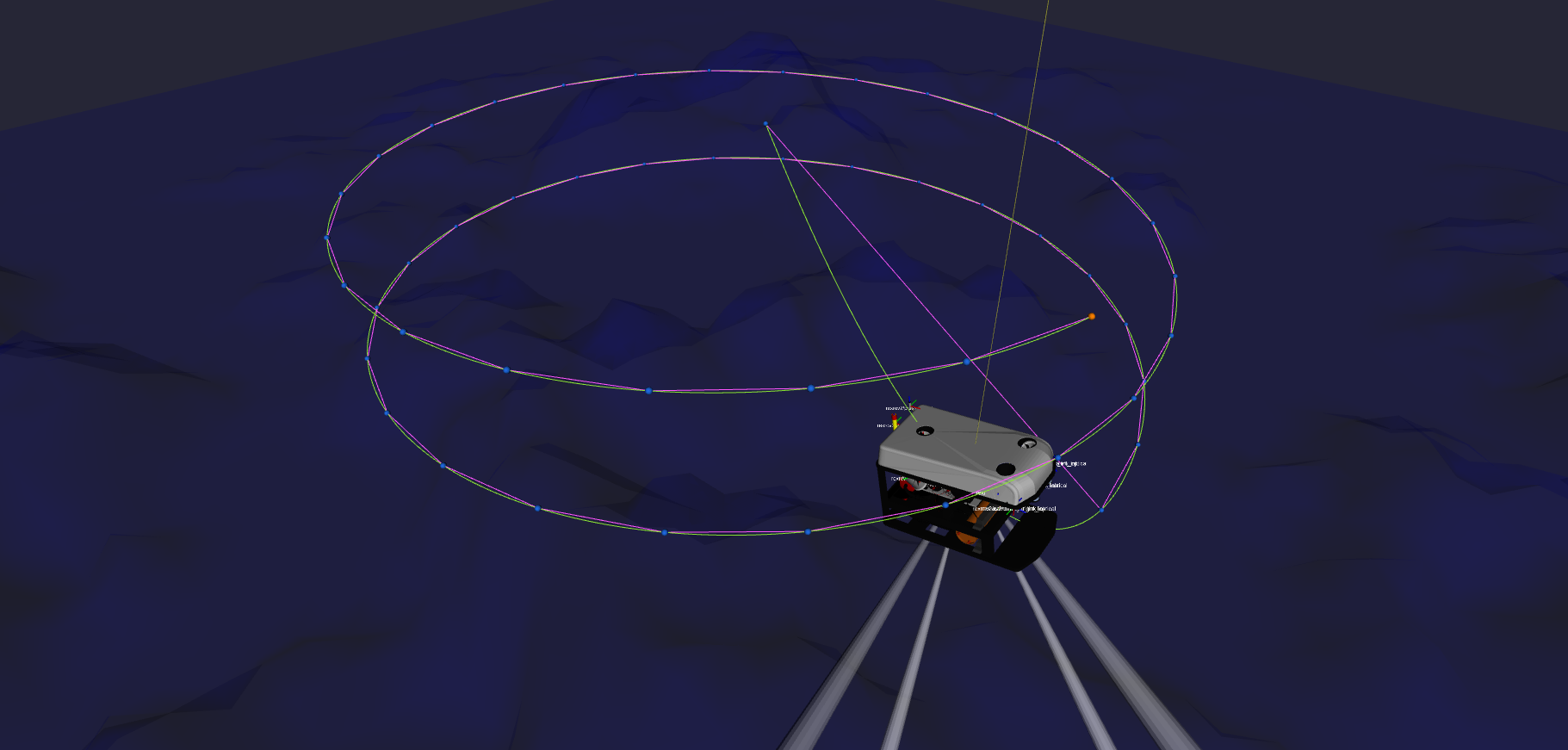###### Bézier curves¶

Bézier curves is a type of parametric curve. The BezierCurve class in uuv_trajectory_generator Python module allows the construction of curves of order 3, 4 or 5.

This segment constructor is used by the cubic interpolator, the linear interpolator with polynomial blends and the final interpolator for the Dubins path generator.

Note

An example of the use of the BezierCurve class from uuv_trajectory_generator Python module can be seen in this Jupyter notebook

##### Path interpolators¶

The path interpolators use a uuv_waypoints.WaypointSet input to compute the path. Velocities and accelerations can also be computed by finite differentiation, if necessary.

###### Linear interpolator¶

The linear interpolator simply connects all the waypoints using line segments.

Warning

This path generator may cause undesired jerk movements around the corners.

Note

An example of the use of the LinearInterpolator class from uuv_trajectory_generator Python module can be seen in this Jupyter notebook

To see a sample of a linear interpolator path being followed by a vehicle, you can start an ROV model with a trajectory tracking controller, such as the following from the rexrov2 package.

roslaunch rexrov2_gazebo start_demo_pid_controller.launch


and then use send its controller a set of sample waypoints using the linear input for the interpolator as follows

roslaunch uuv_control_utils send_waypoints_file.launch uuv_name:=rexrov2 interpolator:=linear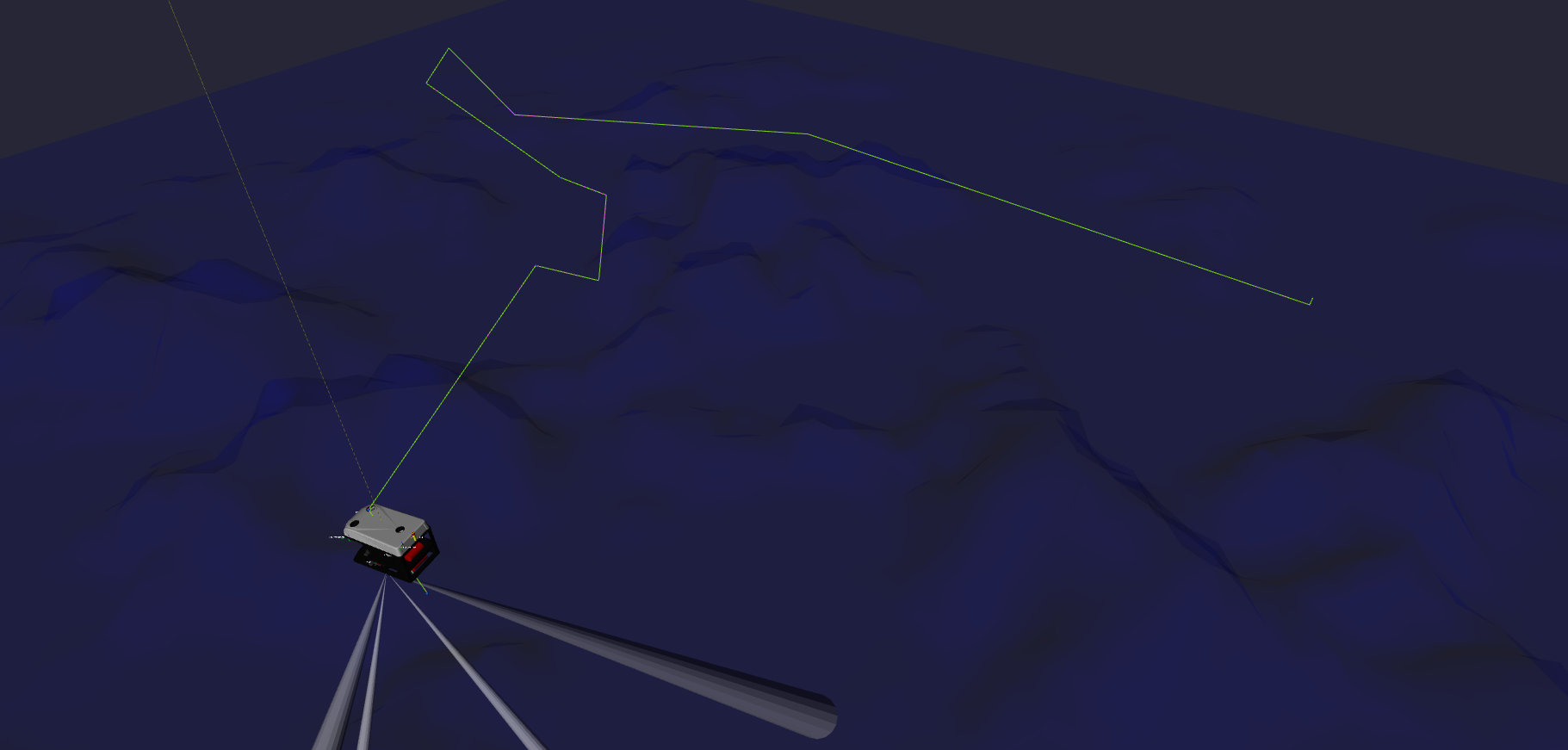###### Cubic interpolator¶

The cubic interpolator computes a cubic Bézier curve that intersepts all the waypoints in the input set.

The algorithm for this interpolator can be found in Biagiotti and Melchiorri, 2008.

Note

Biagiotti, Luigi, and Claudio Melchiorri. Trajectory planning for automatic machines and robots. Springer Science &Business Media, 2008.

Note

An example of the use of the CSInterpolator class from uuv_trajectory_generator Python module can be seen in this Jupyter notebook

To see a sample of a cubic interpolator path being followed by a vehicle, you can start an ROV model with a trajectory tracking controller, such as the following from the rexrov2 package.

roslaunch rexrov2_gazebo start_demo_pid_controller.launch


and then use send its controller a set of sample waypoints using the cubic input for the interpolator as follows

roslaunch uuv_control_utils send_waypoints_file.launch uuv_name:=rexrov2 interpolator:=cubic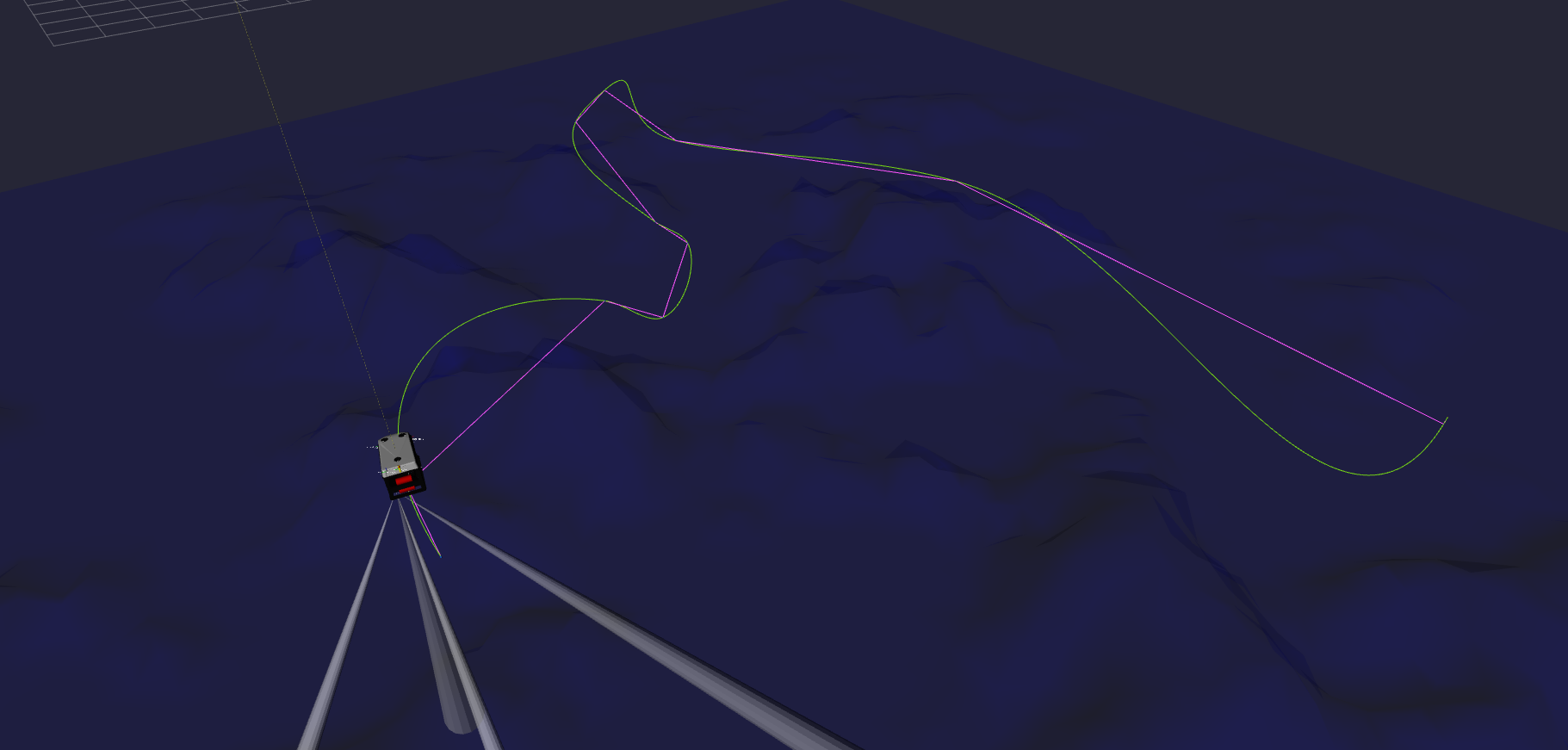###### Linear interpolator with polynomial blends¶

This intepolator is an implementation of the algorithm presented in Biagiotti and Melchiorri, 2008 for trajectory generation of manipulators. It consists basically on line segments generated between each pair of points on the path and Bezier curve of fifth order around the corners.

Note

Biagiotti, Luigi, and Claudio Melchiorri. Trajectory planning for automatic machines and robots. Springer Science &Business Media, 2008.

Note

An example of the use of the LIPBInterpolator class from uuv_trajectory_generator Python module can be seen in this Jupyter notebook

To see an example of this interpolator path being followed by a vehicle, you can start an ROV model with a trajectory tracking controller, such as the following from the rexrov2 package.

roslaunch rexrov2_gazebo start_demo_pid_controller.launch


and then use send its controller a set of sample waypoints using the lipb input for the interpolator as follows

roslaunch uuv_control_utils send_waypoints_file.launch uuv_name:=rexrov2 interpolator:=lipb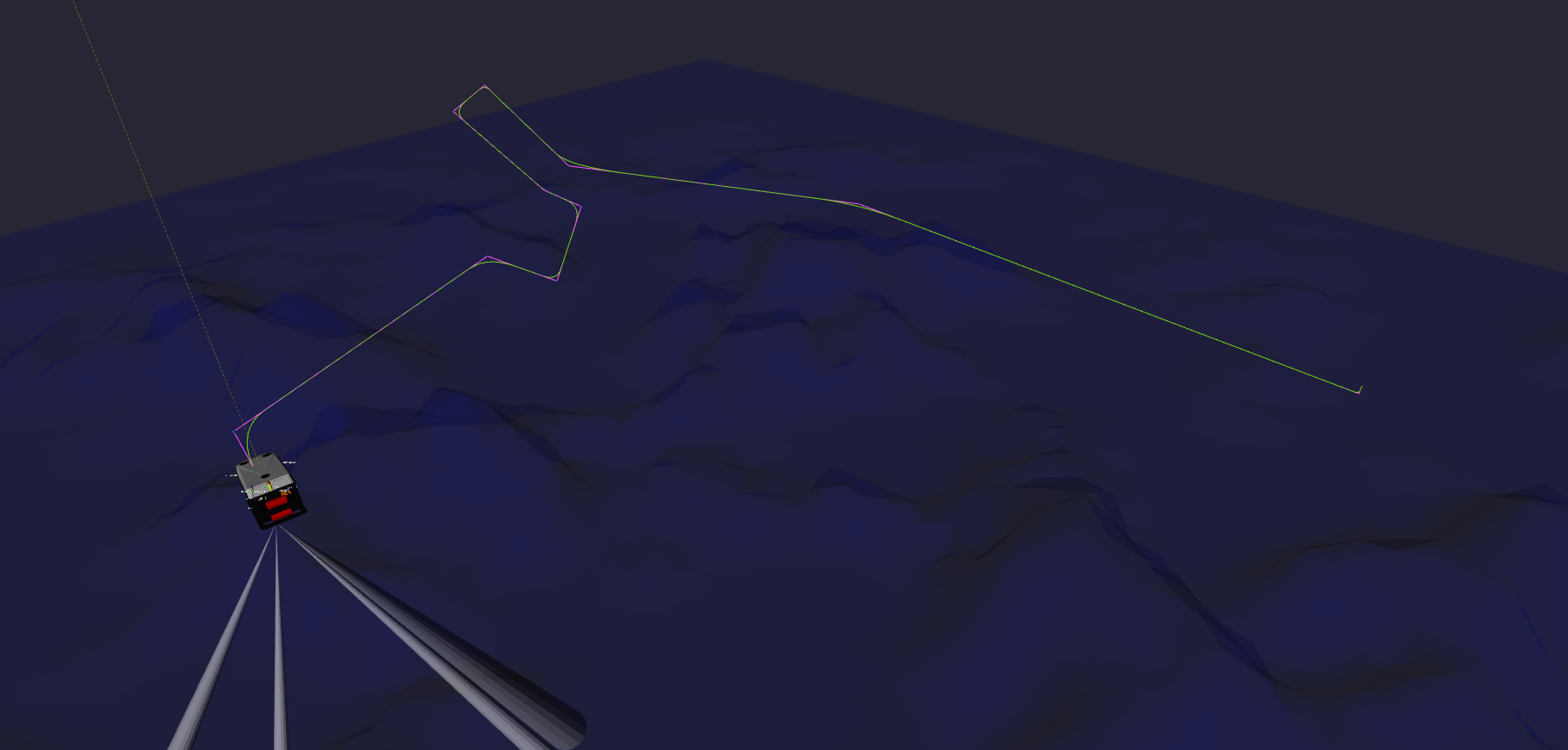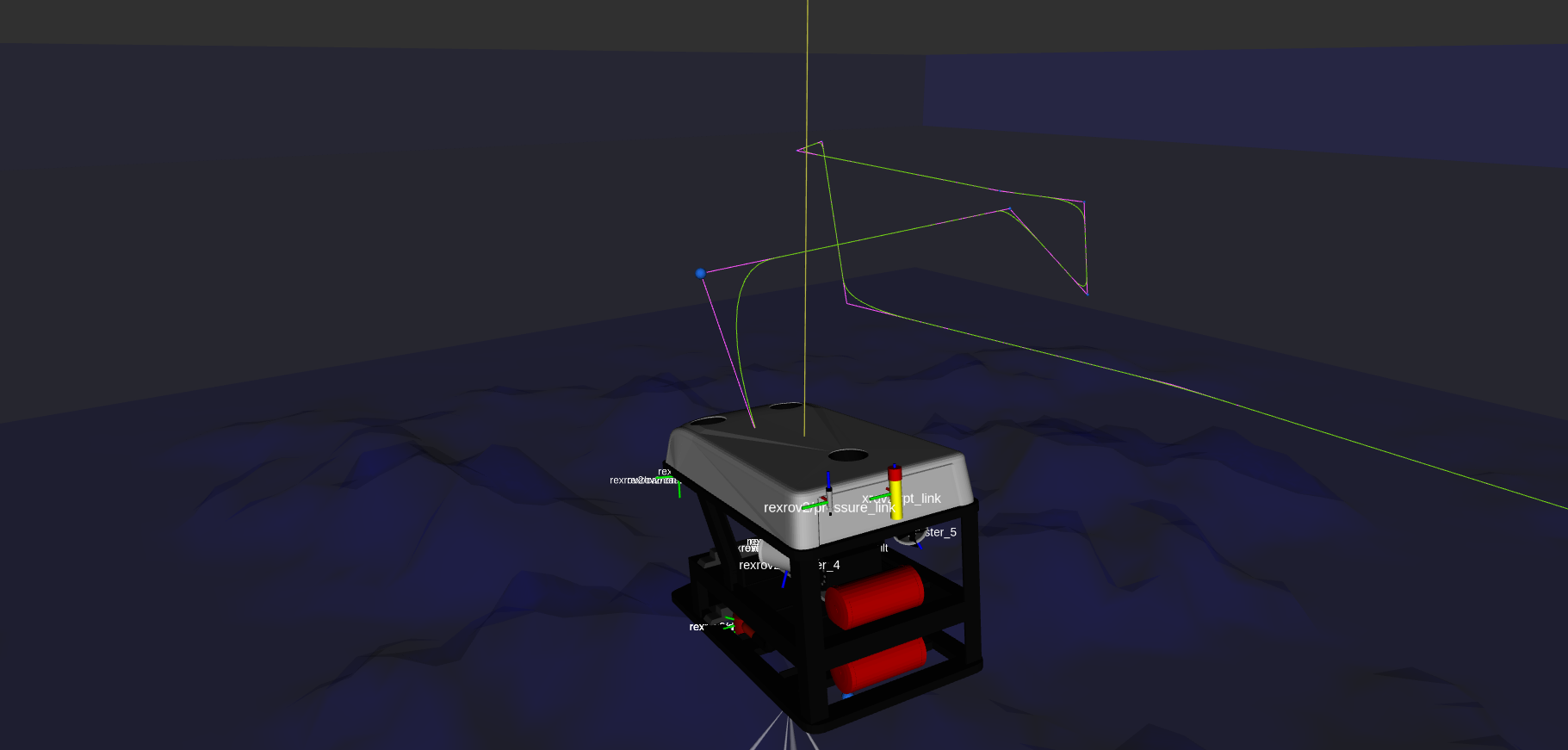###### Dubins interpolator¶

The Dubins path generator is an expansion of the 2D Dubins path algorithm that computes the shortest curve between two points. This path is ideal for vehicles that can only travel forwards, such as AUVs.

Some references for variations the 3D version of the algorithm are Owen et al., 2014, Cai et al., 2017, Hansen et al., 2016 and Lin et al., 2014.

As a safety measure, specially for vehicles such as AUVs that will maneuver using fins, a configurable maximum pitch angle (set by default to 5 degrees) is set to control the behaviour of the climbing of the vehilce. In case the necessary pitch angle between two waypoints exceeds this angle, a helical path is introduced to avoid the generation of pitch set-points that might make the vehicle stall. This will be seen in the example below with the rexrov2 vehicle.

Note

Owen, Mark, Randal W. Beard, and Timothy W. McLain. “Implementing Dubins Airplane Paths on Fixed-Wing UAVs*.” Handbook of Unmanned Aerial Vehicles (2014): 1677–1701.

Cai, Wenyu, Meiyan Zhang, and Yahong Zheng. “Task Assignment and Path Planning for Multiple Autonomous Underwater Vehicles Using 3D Dubins Curves †.” Sensors 17.7 (2017): 1607.

Hansen, Karl D., and Anders La Cour-Harbo. “Waypoint Planning with Dubins Curves Using Genetic Algorithms.” 2016 European Control Conference (ECC) (2016): n. pag.

Lin, Yucong, and Srikanth Saripalli. “Path Planning Using 3D Dubins Curve for Unmanned Aerial Vehicles.” 2014 International Conference on Unmanned Aircraft Systems (ICUAS) (2014): n. pag.

Note

See here a Jupyter notebook on the computation of the 2D Dubins path algorithm, including the computation of all the possible curves between two points.

Note

An example of the use of the DubinsInterpolator class from uuv_trajectory_generator Python module can be seen in this Jupyter notebook

To see an example of this interpolator path being followed by a vehicle, you can start an ROV model with a trajectory tracking controller, such as the following from the rexrov2 package.

roslaunch rexrov2_gazebo start_demo_pid_controller.launch


and then use send its controller a set of sample waypoints using the dubins input for the interpolator as follows

roslaunch uuv_control_utils send_waypoints_file.launch uuv_name:=rexrov2 interpolator:=dubins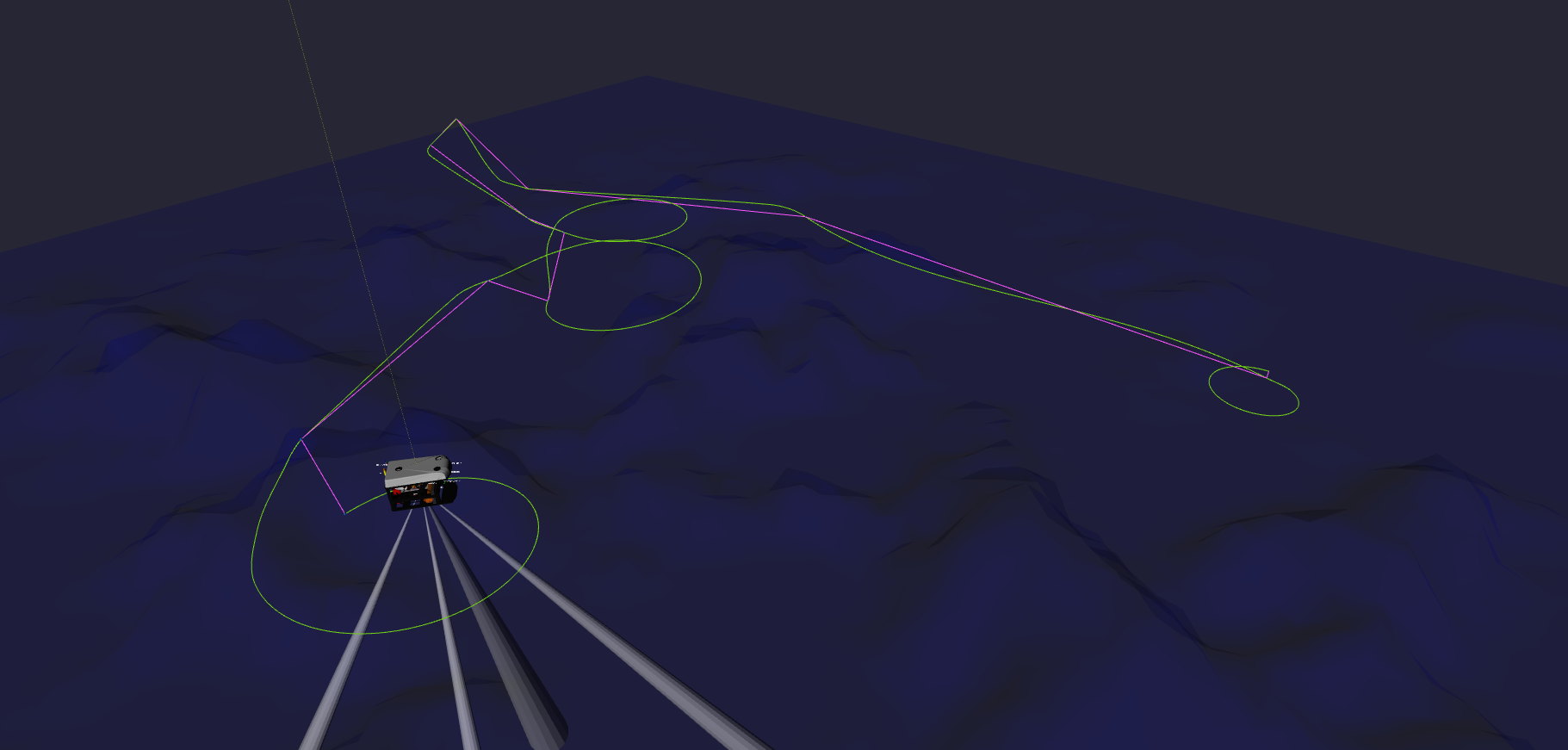Below the helical path is generated between the first two waypoints since the pitch angle exceed the limit.